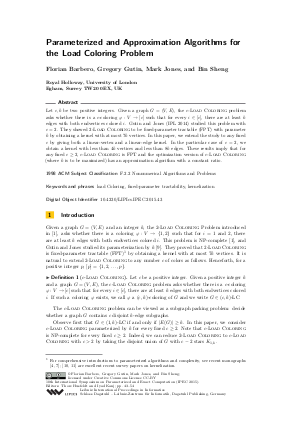Document# Parameterized and Approximation Algorithms for the Load Coloring Problem

### Authors Florian Barbero, Gregory Gutin, Mark Jones, Bin Sheng## File

LIPIcs.IPEC.2015.43.pdf
• Filesize: 495 kB
• 12 pages

## Cite As

Florian Barbero, Gregory Gutin, Mark Jones, and Bin Sheng. Parameterized and Approximation Algorithms for the Load Coloring Problem. In 10th International Symposium on Parameterized and Exact Computation (IPEC 2015). Leibniz International Proceedings in Informatics (LIPIcs), Volume 43, pp. 43-54, Schloss Dagstuhl - Leibniz-Zentrum für Informatik (2015)
https://doi.org/10.4230/LIPIcs.IPEC.2015.43

## Abstract

Let c, k be two positive integers. Given a graph G=(V,E), the c-Load Coloring problem asks whether there is a c-coloring varphi: V => [c] such that for every i in [c], there are at least k edges with both endvertices colored i. Gutin and Jones (IPL 2014) studied this problem with c=2. They showed 2-Load Coloring to be fixed-parameter tractable (FPT) with parameter k by obtaining a kernel with at most 7k vertices. In this paper, we extend the study to any fixed c by giving both a linear-vertex and a linear-edge kernel. In the particular case of c=2, we obtain a kernel with less than 4k vertices and less than 8k edges. These results imply that for any fixed c >= 2, c-Load Coloring is FPT and the optimization version of c-Load Coloring (where k is to be maximized) has an approximation algorithm with a constant ratio.
##### Keywords
• fixed-parameter tractability
• kernelization

## Metrics

• Access Statistics
• Total Accesses (updated on a weekly basis)
0